Figures index

Edeh O.D.F., Ossia C.V.

Journal of Automation and Control. 2019, 7(1), 7-14 doi:10.12691/automation-7-1-2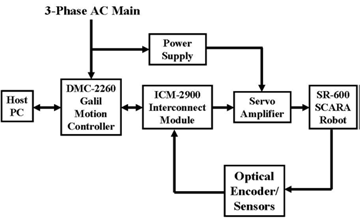• Figure 1. DMC-2260-based SR-600 SCARA Robot Closed-Loop Control System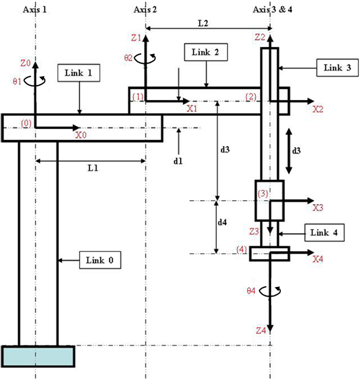• Figure 2. SCARA Robot frame assignments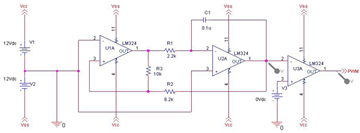• Figure 3. PWM Generator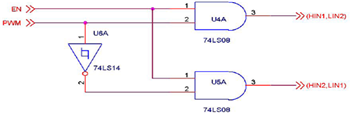• Figure 4. Logic Control Unit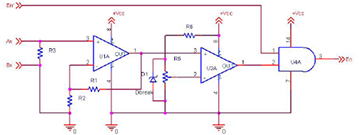• Figure 5. Over-current sense circuit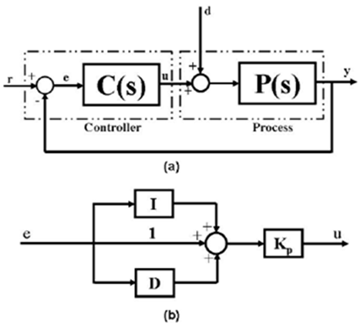• Figure 6. Basic PID for (a) Control Structure, (b) Model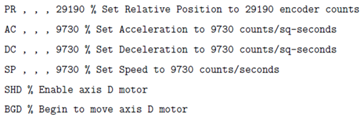• Figure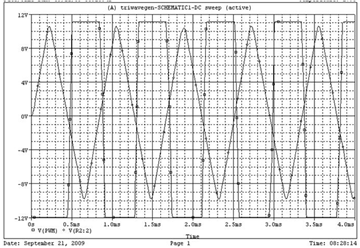• Figure 7. PWM Simulation Result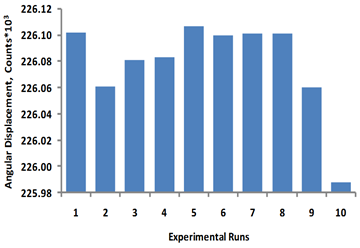• Figure 8. Axis 1 Travel limits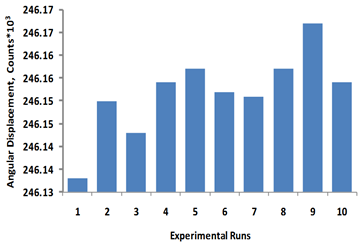• Figure 9. Axis 2 Travel limits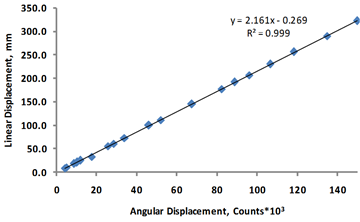• Figure 10. Axis 3 Travel limits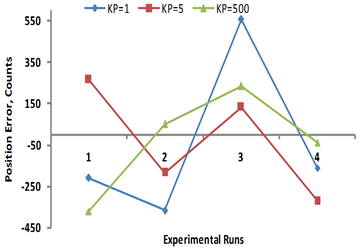• Figure 11. Position errors for constant Ki=Kd=0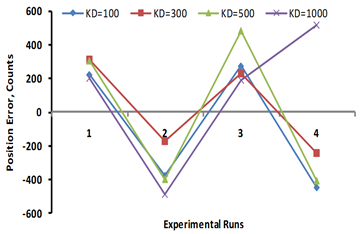• Figure 12. Position errors for constant Ki=0, Kp=50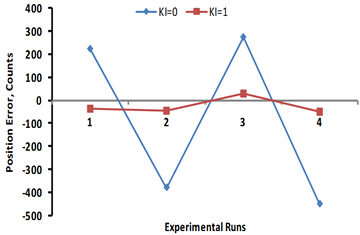• Figure 13. Position errors for constant Kp=50, Kd=100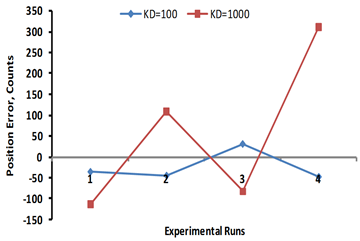• Figure 14. Position errors for constant Ki=1, Kp=50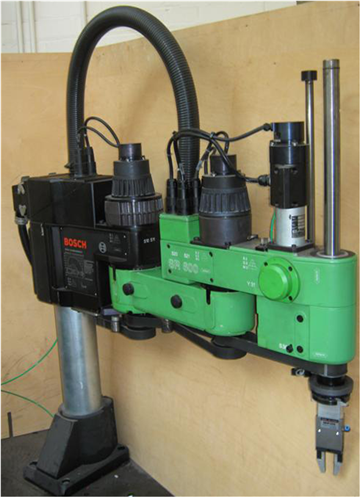• Figure A1. SR-600 Bosch SCARA Robot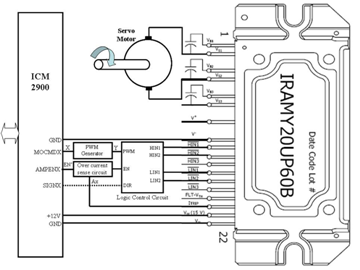• Figure A2. Power Amplifier Circuit